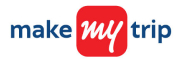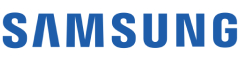New update is available. Click here to update.
Last Updated: 27 Feb, 2021

# Merge Two Sorted Arrays

Moderate+47 more companies

## Problem statement#### For example:

``````‘ARR1’ = [3 6 9 0 0]
‘ARR2’ = [4 10]
After merging the ‘ARR1’ and ‘ARR2’ in ‘ARR1’.
‘ARR1’ = [3 4 6 9 10]
``````
##### Input Format
``````The first line of input contains an integer ‘T’ which denotes the number of test cases or queries to be run. Then the test cases follow.

The first line of each test case contains two space-separated integers ‘M’ and ‘N’, which represent the size of ‘ARR1’ and ‘ARR2’.

The next lines of each test case contain ‘M’ space-separated integers and ‘N’ zeros (i.e. 0) which represent the number of elements in ‘ARR1’.

The next lines of each test case contain ‘N’ space-separated integers which represent the number of elements in ‘ARR2’.
``````
##### Output Format :
``````For each test case, return the ‘ARR1’ after merging.

Print the output of each test case in a separate line.
``````

#### Note:

``````You do not need to print anything; it has already been taken care of. Just implement the given function.
``````
##### Constraints:
``````1 <= ‘T’ <= 100
1 <= ‘M’, ‘N’ <= 5000
1 <= ‘ARR1[i], ARR2[i]’ <= 100000

Time Limit: 1 second
``````## Approaches

### 01 ApproachOur main task is that after merging ‘ARR2’ into ‘ARR1’. The resultant ‘ARR1’ is also sorted. So first we simply add all the elements of ‘ARR2’ into ‘ARR1’. Then we can apply any sorting algorithm to sort ‘ARR1’

#### Algorithm:

1. We run a loop for ‘i’ = 0 to ‘N’:
• ‘ARR1[M + i]’ = ‘ARR2[i]’
2. Sort the array/list ‘ARR1’.
3. Finally, return ‘ARR1’.